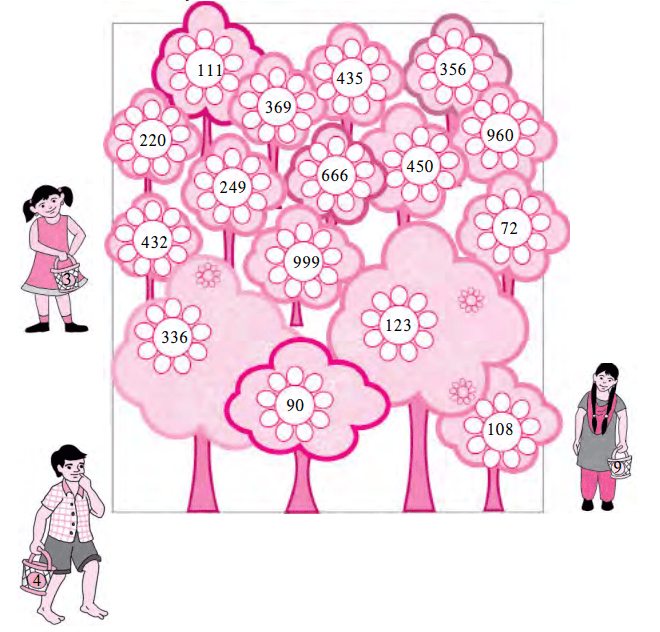# Maharashtra Board Class 6 Maths Solutions Chapter 8 Divisibility Practice Set 22

## Maharashtra State Board Class 6 Maths Solutions 8 Divisibility Practice Set 22

Question 1.
There are some flowering trees in a garden. Each tree bears many flowers with the same number printed on it. Three children took a basket each to pick flowers. Each basket has one of the numbers, 3, 4 or 9 on it. Each child picks those flowers which have numbers divisible by the number on his or her basket. If He / She takes only 1 flower from each tree. Can you tell which numbers the flowers in each basket will have?Solution:
Each child will have flowers bearing the following numbers:
Girl with basket number 3: 111, 369, 435, 249, 666, 450, 960, 432, 999, 72, 336, 90, 123, 108
Boy with basket number 4: 356, 220, 432, 960, 72, 336, 108
Girl with basket number 9: 369, 666, 450, 432, 999, 72, 90, 108

#### Maharashtra Board Class 6 Maths Chapter 8 Divisibility Practice Set 22 Intext Questions and Activities

Question 1.
Read the numbers given below. Which of these numbers are divisible by 2, by 5, or by 10? Write them in the empty boxes. 125,364,475,750,800,628,206,508,7009,5345,8710. (Textbook pg. no. 43)

 Divisible by 2 Divisible by 5 Divisible by 10

Solution:

 Divisible by 2 Divisible by 5 Divisible by 10 364,750, 800, 628, 206, 508, 8710 125,475, 750, 800, 5345, 8710 750, 800, 8710

Question 2.
Complete the following table: (Textbook pg. no. 43)

 Number Sum of digits in the number Is the sum divisible by 3? Is the given number divisible by 3? 63 6 + 3 = 9 ✓ ✓ 872 17 X X 91 552 9336 4527

Solution:

 Number Sum of digits in the number Is the sum divisible by 3? Is the given number divisible by 3? 63 6 + 3 = 9 ✓ ✓ 872 8 + 7 + 2 = 17 X X 91 9 + 1 = 10 X X 552 5 + 5 + 2 = 12 ✓ ✓ 9336 9 + 3 + 3 + 6 = 21 ✓ ✓ 4527 4 + 5 + 2 + 7 = 18 ✓ ✓

Question 3.
Complete the following table: (Textbook pg. no. 44)

 Number Divide the number by 4. Is it completely divisible? The number formed by the digits in the tens and units places. Is this number divisible by 4? 992 ✓ 92 ✓ 7314 6448 8116 7773 3024

Solution:

 Number Divide the number by 4. Is it completely divisible? The number formed by the digits in the tens and units places. Is this number divisible by 4? 992 ✓ 92 ✓ 7314 X 14 X 6448 ✓ 48 ✓ 8116 ✓ 16 ✓ 7773 X 73 X 3024 ✓ 24 ✓

Question 4.
Complete the following table: (Textbook pg. no. 44)

 Number Divide the number by 9. Is it completely divisible? Sum of the digits in the number. Is the sum divisible by 9? 1980 ✓ 1 + 9 + 8 + 0 = 18 ✓ 2999 X 2 + 9 + 9 + 9 = 29 X 5004 13389 7578 69993

Solution:

 Number Divide the number by 9. Is it completely divisible? Sum of the digits in the number. Is the sum divisible by 9? 1980 ✓ 1 + 9 + 8 + 0 = 18 ✓ 2999 X 2 + 9 + 9 + 9 = 29 X 5004 ✓ 5 + 0 + 0 + 4 = 9 ✓ 13389 X 1 + 3 + 3 + 8 + 9 = 24 X 7578 ✓ 7 + 5 + 7 + 8 = 27 ✓ 69993 ✓ 6 + 9 + 9 + 9 + 3 = 36 ✓